# Capital Structure – Financial Management MCQ

Capital Structure – CS Executive Financial and Strategic Management MCQ Questions with Answers you can quickly revise the concepts.

## Capital Structure – Financial Management MCQ

Question 1.
…………. refers to the mix of a firm’s capitalization and includes long term sources of funds.
(A) Leverage
(B) Capital structure
(C) Debt mix
(D) Owner’s equity
(B) Capital structureQuestion 2.
The term “capital structure” refers to:
(A) Current assets & current liabilities
(B) Long-term debt, preferred stock, and common stock equity
(C) Total assets minus liabilities
(D) Share holders’ equity
(B) Long-term debt, preferred stock, and common stock equityQuestion 3.
The decisions regarding the forms of financing, their requirements and their relative proportions in total capitalization known as –
(A) Equity decisions
(B) Equilibrium decisions
(C) Outright decisions
(D) Capital structure decisions
(D) Capital structure decisions

Question 4.
Which of the following statement is false?
I. In case the firm wants to grow at a faster pace, it would be required to incorporate debt in its capital structure to a greater extent.
II. If the firm has no long term debt in its capital structure, it means that either it is risk averse or it has cost of equity capital or cost of retained earnings less than the cost of debt.
Select the correct answer from the options given below:
(A) Statement I is true while Statement II is false.
(B) Statement I is false while Statement II is true.
(C) Both Statement I and Statement II are false.
(D) Both Statement I and Statement II are true.
(D) Both Statement I and Statement II are true.

Question 5.
While designing a capital structure a finance manager should choose a pattern of capital which –
(A) Minimizes cost of capital
(B) Maximizes the owners return.
(C) Maximizes cost of capital and minimizes the owners return.
(D) Both (A) and (B)
(D) Both (A) and (B)

Question 6.
Which of the following changes in capital structure would you recommend for growth at faster rate?
(A) Incorporate more retained earnings out of profit and loss account.
(B) Incorporate debt in its capital structure to a greater extent.
(C) Merge with other companies.
(D) Pay more dividend to equity share-holders.
(B) Incorporate debt in its capital structure to a greater extent.

Question 7.
The manner in which an organization’s assets are financed is referred to as its –
(A) Capital structure
(B) Financial structure
(C) Asset structure
(D) Owners structure
(B) Financial structure

Question 8.
Optimal capital structure consists of -…………..
(A) Appropriate mix of fixed assets and current assets.
(B) Appropriate mix of long term debts and fixed assets.
(C) Appropriate mix of sales and profit.
(D) Appropriate mix of debt and equity.
(D) Appropriate mix of debt and equity.

Question 9.
Which of the following is not included in capital structure?
(A) Long term debt
(B) Preferred stock
(C) Current assets
(D) Retained earnings
(C) Current assets

Question 10.
Which of the following shows significance of capital structure?
(A) Capital structure reflects the overall strategy of the firm.
(B) One can get a reasonably accurate broad idea about the risk profile of the firm from its capital structure.
(C) The capital structure acts as a tax management tool.
(D) All of the above
(D) All of the above

Question 11.
Financial structure involves creation of –
(1) Long term assets
(2) Short term assets
Select the correct answer from the options given below:
(A) (2) only
(B) Neither (1) nor (2)
(C) (1) only
(D) Both (1) and (2)
(D) Both (1) and (2)

Question 12.
Which of the following statement is incorrect?
(1) High debt funds in capital structure increases EPS.
(2) High debt funds increases the operating or business risk.
Select the correct answer from the options given below:
(A) Both Statement 1 and Statement 2 are correct.
(B) Statement 1 is correct while Statement 2 is incorrect.
(C) Statement 2 is correct while Statement 1 is incorrect.
(D) Both Statement 1 and Statement 2 are incorrect.
(D) Both Statement 1 and Statement 2 are incorrect.

Question 13.
Financial structure is ……………. concept while capital structure is concept
(A) inappropriate; appropriate
(B) appropriate; inappropriate

Question 14.
Assertion (A):
The capital structure should be determined within the debt capacity of the company and this capacity should not be exceeded.
Reason (R):
The debt capacity of a company depends on its ability to generate future cash flows. It should have enough cash to pay creditors’ fixed charges and principal sum.
Select the correct answer from the options given below:
(A) A is true but R is false
(B) A is false but R is true.
(C) Both A and R are true but R is not correct explanation of A.
(D) Both A and Rare true and R is correct explanation of A.
(D) Both A and Rare true and R is correct explanation of A.

Question 15.
Which of the following capital structure consist of zero debt components in the structure mix?
(A) Pyramid Shaped Capital Structure
(B) Inverted Pyramid Shaped Capital Structure
(C) Horizontal Capital Structure
(D) Vertical Capital Structure
(C) Horizontal Capital Structure

Question 16.
Which of the following statement is false?
(A) The use of excessive debt threatens the solvency of the company.
(B) A firm having operating loss would find it worthwhile to incorporate debt in the capital structure in a greater measure.
(C) The capital structure should be flexible.
(D) None of the above
(B) A firm having operating loss would find it worthwhile to incorporate debt in the capital structure in a greater measure.

Question 17.
One can get a reasonably accurate broad idea about the risk profile of the firm from its –
(A) Dividend policy
(B) Capital structure
(C) Debt service ratio
(D) Earning yield
(B) Capital structure

Question 18.
A critical assumption of the net operating income (NOI) approach to valuation is that:
(A) Debt and equity levels remain unchanged.
(B) Dividends increase at a constant rate.
(C) Ko remains constant regardless of changes in leverage.
(D) Interest expense and taxes are included in the calculation.
(C) Ko remains constant regardless of changes in leverage.

Question 19.
If the debt component in the capital structure is predominant –
(A) The fixed interest cost of the firm will be minimum thereby decreasing its risk.
(B) Earnings per share (EPS) will be very low.
(C) Dividend expectations of equity shareholders are also and P/E Ratio may decrease.
(D) The fixed interest cost of the firm increases thereby increasing its risk.
(D) The fixed interest cost of the firm increases thereby increasing its risk.

Question 20.
Capital structure relates to …………. capital deployment for creation of ……… assets.
(A) long term; long term
(B) long term; short term
(C) short term; long term
(D) short term; short term
(A) long term; long term

Question 21.
Assertion (A):
The capital structure acts as a tax management tool also.
Reason (R):
Relatively lesser component of equity capital is vulnerable to hostile takeovers.
Select the correct answer from the options given below:
(A) A is true but R is false
(B) A is false but R is true.
(C) Both A and R are true but R is not correct explanation of A.
(D) Both A and Rare true and R is correct explanation of A.
(C) Both A and R are true but R is not correct explanation of A.

Question 22.
Select which of the following statement is correct.
Horizontal capital structure -…………
1. is quite stable.
2. is formed by a small amount of equity share capital.
3. there is absence of debt.
4. have increasing component of debt.
Select the correct answer from the options given below:
(A) 1, 2 & 4
(B) 2 & 3
(C) 1 only
(D) 1 & 4 only
(C) 1 only

Question 23.
One can design capital structure with proper proportions of equity, preference and debt mix. The choice of the combination of these sources is called –
(A) Structural mix
(B) Policy mix
(C) Capital structure mix
(D) Finance mix
(C) Capital structure mix

Question 24.
In horizontal capital structure –
(A) expansion of the firm takes place by issuance of debt securities.
(B) expansion of the firm takes place by issuance of debt securities and preferred stocks.
(C) expansion of the firm takes in a lateral manner, i.e. through equity or retained earning only.
(D) expansion of the firm takes place by issuance of short term and marketable securities.
(C) expansion of the firm takes in a lateral manner, i.e. through equity or retained earning only.

Question 25.
According to Cost Principle an ideal pattern or capital structure is one that -…………..
(A) Minimizes cost of capital structure
(B) Maximizes earnings per share (EPS).
(C) Both (A) and (B)
(D) None of the above
(C) Both (A) and (B)

Question 26.
In a …………. the base of the structure is formed by a small amount of equity share capital. This base serves as the foundation on which the super structure of preference share capital and debt is built.
(A) horizontal capital structure
(B) vertical capital structure
(C) diagonal capital structure
(D) matrix capital structure
(B) vertical capital structure

Question 27.
According to Risk Principle……..
(A) Reliance is placed on excessive use of debt financing for capital requirements than common equity.
(B) Reliance is placed on ability of finance manager than external analyst.
(C) Reliance is placed more on common equity for financing capital requirements than excessive use of debt.
(D) Reliance is placed more on short term finance for financing capital requirements than excessive use of working capital.
(C) Reliance is placed more on common equity for financing capital requirements than excessive use of debt.

Question 28.
Match List I with List II: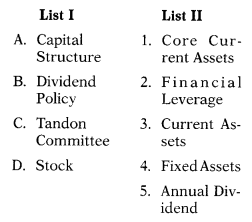Select the correct answer from the options given below(D)

Question 29.
Use of more and more debt and preference capital –
(A) affects equity share values and in unfavourable situation equity share prices may consequently drop.
(B) increases value of debt and preference capital and equity share.
(C) Increases the profit after tax (PAT) even though sales pattern shows decreasing trends.
(D) All of the above
(A) affects equity share values and in unfavourable situation equity share prices may consequently drop.

Question 30.
Match List – I with List – II: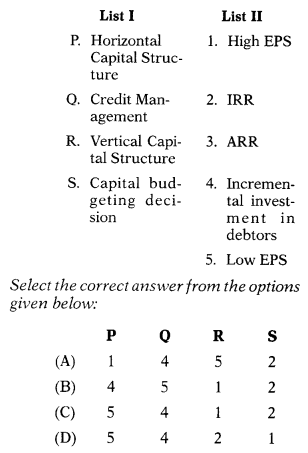(C)

Question 31.
(A) Avoidable risk
(B) Unavoidable risk
(C) Not relevant
(D) Less important than financial risk
(B) Unavoidable risk

Question 32.
Which of the following statement is true in relation to vertical capital structure?
(A) The incremental addition in the capital structure is almost entirely in the form of debt.
(B) The absence of debt it results in the lack of financial leverage and hence low financial risk.
(C) Since there is more equity shares EPS is likely to be high.
(D) For this capital structure combined leverage is very low as compared to other firms in industry which have more equity finance.
(A) The incremental addition in the capital structure is almost entirely in the form of debt.

Question 33.
The rate of tax affects the –
(A) Cost of retained earning
(B) Cost of debt
(C) Cost of equity
(D) All of the above
(D) All of the above

Question 34.
A pyramid shaped capital structure has –
(A) Retained earnings of the firm which are usually lower than the cost of debt.
(B) A large proportion consisting of equity capital and retained earnings which have been ploughed back into the firm over a considerably large period of time.
(C) Incremental addition in the capital structure is almost entirely in the form of debt.
(D) Both (A) and (B)
(D) Both (A) and (B)

Question 35.
Assertion A:
While making a choice of the capital structure the future cash flow position should be kept in mind.
Reason R:
Debt capital should be used only if the cash flow position is really good because a lot of cash is needed in order to make payment of interest and refund of capital.
Select the correct answer from the options given below:
(A) A is true and R is false
(B) A is false and R is true
(C) Both A and R are true and R is not correct explanation of A.
(D) Both A and Rare true and R is correct explanation of A.
(D) Both A and Rare true and R is correct explanation of A.

Question 36.
To have optimal capital structure the firm must fulfil the following conditions:
I. Return on investment should be greater than cost of investment.
II. There should be minimum financial risk.
III. There is absence of equity finance.
IV. The capital structure should be flexible
V. Cost of investment should be greater than ROI.
Select correct answer from the options given below:
(A) III, I
(B) IV,II & V
(C) II, I & IV
(D) II & IV
(C) II, I & IV

Question 37.
Business risk is influenced by –
(A) Revenue
(B) Variable cost
(C) Fixed assets
(D) All of the above
(D) All of the above

Question 38.
Capital Structure of a firm –
(A) Is a reflection of the overall investment and financing strategy of the firm.
(B) Shows how much reliance is being placed by the firm on external sources of finance and how much internal accrual is being used to finance expansions
(C) Means the structure or constitution or break-up of the capital employed by a firm.
(D) All of the above
(D) All of the above

Question 39.
With the help of Interest Coverage Ratio (ICR) ratio an effort is made to find out –
(A) How many times the profit after tax (PAT) is available to the payment of interest.
(B) How many times the net operating profit after tax (NOPAT) is available to the payment of interest.
(C) How many times the EBIT is available to the payment of interest.
(D) Most suitable bank for negotiation.
(C) How many times the EBIT is available to the payment of interest.

Question 40.
Pyramid Shaped Capital Structure –
(A) Have a high proportion of fixed assets and considerably a very low proportion of current assets.
(B) Have a high proportion of debts and considerably a very low proportion of equity.
(C) Have a high proportion of equity and considerably a very low proportion of debt.
(D) Have a high proportion of current assets and considerably a very’ low proportion of liquid assets.
(C) Have a high proportion of equity and considerably a very low proportion of debt.

Question 41.
Financial Risk is –
(A) Affected by demand of firm products, variations in prices and proportion of fixed cost in total cost.
(B) Represented by the variability of earnings before interest and tax (EBIT)
(C) Is unavoidable if firm does not use debt in its capital structure.
(D) All of the above
(C) Is unavoidable if firm does not use debt in its capital structure.

Question 42.
Inverted Pyramid Shaped Capital Structure –
(A) Has a large component of equity capital.
(B) Is highly stable and permanent.
(C) Is opposite as that of pyramid shaped capital structure.
(D) Has reasonable level of debt but an ever increasing component of retained earnings.
(C) Is opposite as that of pyramid shaped capital structure.

Question 43.
Which of the following statement is true?
(0 Flexibility principle states that the management chooses such a combination of sources of financing which it finds easier to adjust according to changes in need of funds in future too.
(ii) Penalty for not meeting financial obligations is bankruptcy.
(iii) Firms with high business risk therefore tend toward less highly leveraged capital structures, and firm with low business risk tend toward more highly leveraged capital structures.
Select correct answer from the options given below:
(A) (i) only
(B) (i) and (iii)
(C) (i) only
(D) All of the above
(D) All of the above

Question 44.
Which of the following is vulnerable to hostile takeovers?
(A) Horizontal Capital Structure
(B) Vertical Capital Structure
(C) Pyramid Shaped Capital Structure
(D) All of the above
(B) Vertical Capital Structure

Question 45.
Floatation costs are those expenses which are incurred while –
(A) Issuing securities
(B) Repayment of debts
(D) Repayment of equity and debts
(A) Issuing securities

Question 46.
Operating/Business Risk refers to the risk of –
(A) Inability to pay fixed financial payments (e.g., payment of interest, preference dividend, return of the debt capital, etc.)
(B) Inability to discharge permanent operating costs (e.g., rent of the building, payment of salary, insurance instalment, etc.)
(C) Both (A) and (B)
(D) None of the above
(B) Inability to discharge permanent operating costs (e.g., rent of the building, payment of salary, insurance instalment, etc.)

Question 47.
Which of the following is floatation cost?
(A) Commission of underwriters
(B) Brokerage paid on issue of securities
(C) Stationery expenses on issue of securities
(D) All of the above
(D) All of the above

Question 48.
……….. denotes the level of EBIT for which the firm’s EPS equals zero.
(A) Financial break-even point
(B) Margin of safety
(C) Equilibrium point
(D) Min-max point
(A) Financial break-even point

Question 49.
Which of the following is correct formula to calculate EPS?
(A) [(EBIT + I) (1 – T) – Dp]/N0
(B) [EBIT -1 + T – Dp]/N0
(C) [(EBIT -1) (1 – T) – Dp]/N0
(D) [(EBIT -1) (1 – T) + Dp]/N0
(C) [(EBIT -1) (1 – T) – Dp]/N0

Question 50.
If the EBIT is less than the financial 5 breakeven point, then the EPS will be –
(A) Positive
(B) Negative
(C) Zero
(D) Maximum
(B) Negative

Question 51.
According to Net Income Approach, capital structure decision –
(A) Is relevant to the value of the firm.
(B) Of the firm are irrelevant.
(C) Will not lead to any change in the total value of the firm and the market price of shares.
(D) Division between debt and equity is irrelevant.
(A) Is relevant to the value of the firm.

Question 52.
According to Net Operating Income Approach –
(A) Capital structure decisions of the firm are irrelevant.
(B) Any change in the leverage will not lead to any change in the total value of the firm and the market price of shares, as the overall cost of capital is independent of the degree of leverage.
(C) The division between debt and equity is irrelevant.
(D) All of the above
(D) All of the above

Question 53.
According to ……………, the firm can increase its total value by decreasing its overall cost of capital through increasing the degree of leverage.
(A) Net Operating Income Approach
(B) Net Income Approach
(C) Both (A) and (B)
(D) Neither (A) nor (B)
(B) Net Income Approach

Question 54.
As per Net Income Approach the value of the firm will be maximum at a point where –
(A) Average cost of equity is minimum.
(B) Average cost of debt is minimum.
(C) Weighted average cost of equity is maximum.
(D) Weighted average cost of capital is minimum.
(D) Weighted average cost of capital is minimum.

Question 55.
Any change in the leverage will not lead to any change in the total value of the firm and the market price of shares, as the overall cost of capital is independent of the degree of leverage. This is as per
(A) Net Operating Income Approach
(B) Net Income Approach
(C) Both (A) and (B)
(D) Neither (A) nor (B)
(A) Net Operating Income Approach

Question 56.
Inability to pay fixed financial payments e.g. payment of interest, preference dividend, return of the debt capital, etc. is called as –
(B) Financial risk
(C) Operating risk
(D) (A) and (C)
(B) Financial risk

Question 57.
If expected level of EBIT is more than the breakeven point, then the EPS will be –
(A) Minimum
(B) Negative
(C) Positive
(D) Infinite
(C) Positive

Question 58.
The overall cost of capital under Net Income Approach is –
(A) EBIT 4 ÷ Value of firm
(B) Net Income 4÷ Ke
(C) Value of firm 4 ÷ EBIT
(D) EBIT4 ÷ Ko
(A) EBIT 4 ÷ Value of firm

Question 59.
Which formula would you use to calculate market value of equity?
(A) Net Income 4÷Ke
(B) Ke ÷ Net Income
(C) Sales 4÷Ke
(D) Gross Profit 4÷ Ke
(A) Net Income 4÷Ke

Question 60.
Which of the following proposition is made by Modigliani and Miller?
(A) The total market value of a firm and its cost of capital are independent of its capital structure.
(B) The cost of equity (Ke) is equal to capitalization rate of pure equity stream plus a premium for financial risk.
(C) The cut-off rate for investment decision making for a firm in a given risk class is not affected by the manner in which the investment is financed.
(D) All of the above
(D) All of the above

Question 61.
Which of the following assumption is valid as per MM Approach?
1. There is imperfect competition in the market.
2. There is no transaction cost.
3. All investors are rational.
4. All information is not freely available. Select the correct answer from the options given below:
(A) 1 & 2
(B) 4 & 1
(C) 1 & 3
(D) 3 & 2
(D) 3 & 2

Question 62.
According Modigliani & Miller Approach
(A) Individuals (arbitragers) through the use of personal leverage can alter corporate leverage.
(B) Financial risk increases with more debt content in the capital structure.
(C) The total value of a firm is not affected by its capital structure
(D) All of the above
(D) All of the above

Question 63.
A situation where a firm has more capital than it needs is called as –
(A) Over Finance
(B) Over Capitalization
(D) Over Realization
(B) Over Capitalization

Question 64.
Which of the following is one of the causes of over capitalization?
(A) Reduction in the market price of shares.
(B) Borrowing huge amount at higher rate than rate at which company can earn.
(C) Reduction in the rate of dividend and interest payments.
(D) Buying of shares in the unleveraged firm.
(B) Borrowing huge amount at higher rate than rate at which company can earn.

Question 65.
Which of the following is one of the causes of over capitalization?
(A) Raising more money through issue of shares or debentures than company can employ profitably.
(B) Excessive payment for the acquisition of fictitious assets such as goodwill etc.
(C) Improper provision for depreciation, replacement of assets and distribution of dividends at a higher rate.
(D) All of the above
(D) All of the above

Question 66.
The pecking order theory is popularized by-
(A) Franco and Merton
(B) Modigliani and Miller
(C) Myers and Majluf
(D) Myers and Merton
(C) Myers and Majluf

Question 67.
Financing comes from three sources, internal funds, debt and new equity. Companies prioritize their sources of financing, first preferring internal financing, and then debt, lastly raising equity as a “last resort”. Hence, internal financing is used first; when that is depleted, then debt is issued; and when it is no longer sensible to issue any more debt, equity is issued. This is as per
(A) Order pecking theory
(B) Sequential theory
(C) Sequential pecking theory
(D) Pecking order theory
(D) Pecking order theory

Question 68.
Finance function comprises –
(A) Safe custody of funds only
(B) Expenditure of funds only
(C) Procurement of finance only
(D) Procurement & effective use of funds
(D) Procurement & effective use of funds

Question 69.
Finance functions includes –
(A) Planning for funds
(B) Raising of funds
(C) Allocation of resources
(D) All of the above
(D) All of the above

Question 70.
Earning Yield computed by
(A) EPS/Current Market Price Per Share
(B) Paid up value of Share/100
(C) EPS/Profit × 100
(D) EPS/Market Price
(A) EPS/Current Market Price Per Share

Question 71.
Assertion (A):
High capital gearing leads to greater speculation.
Reason (R):
Proportion of equity share capital in relation to the total capital comprising the other securities is small leading to capitalization being highly geared.
(A) Both A and R are true and R is the correct explanation of A.
(B) Both A and R are true but R is not a correct explanation of A.
(C) A is true but R is false
(D) A is false but R is true
(D) A is false but R is true

Question 72.
Which is external source of finance?
(A) Letters of Credit
(C) Finance from Companies
(D) All of the above
(D) All of the above

Question 73.
The traditional approach towards the valuation of a company assumes that –
(A) The cost of capital is independent of the capital structure of the firm.
(B) The firm maintains constant risk regardless of the type of financing employed.
(C) There exists no optimal capital structure.
(D) That management can increase the total value of the firm through the judicious use of financial leverage.
(D) That management can increase the total value of the firm through the judicious use of financial leverage.

Question 74.
Which of the following statements regarding the net operating income approach is incorrect?
(A) The overall capitalization rate, Ko is constant.
(B) The cost of debt funds, K is constant.
(C) The required return on equity, Kc, is constant.
(D) The total value of the firm is unaffected by changes in financial leverage.
(C) The required return on equity, Kc, is constant.

Question 75.
Two identical companies exist except that Company A uses no debt and Company B uses some debt. The total value of Company A is less than the total value of Company B, but you own 2% of Company B. Based on the arguments by Modigliani and Miller regarding the total value principle, what should you do?
(A) Buy 2% of Company A with funds from “shorting” your shares in Company B. Submit a press release that
the two companies should be worth identical values. This will cause Company A to rise in value and leave you extra funds for investment.
(B) You should borrow enough funds to equal the difference in company value, purchase shares of Company A with these funds, and sell your shares in Company B. This will leave extra funds for an investment of your choice.
(C) Sell your shares, personally borrow 2% of the quantity of company debt, and purchase 2% of Company A. This will leave extra funds for an investment of your choice.
(D) Sell enough of your shares (Company B) to pin-chase 2% of Company A. This will leave extra funds for an investment of your choice.
(C) Sell your shares, personally borrow 2% of the quantity of company debt, and purchase 2% of Company A. This will leave extra funds for an investment of your choice.

Question 76.
Which term would most likely be associated with the phrase “actions speak louder than words”?
(A) Incentive signalling
(B) Shareholder wealth maximization
(C) Financial signalling
(D) Optimal capital structure
(C) Financial signalling

Question 77.
External sources of finance do not include:
(A) Overdrafts
(B) Leasing
(C) Retained earnings
(D) Debentures
(C) Retained earnings

Question 78.
Internal sources of finance do not include:
(A) Retained earnings
(B) Ordinary shares
(C) Better management of working capital
(B) Ordinary shares

Question 79.
A firm’s optimal capital structure:
(A) Is the debt-equity ratio that exists at the point where the firm’s weighted after-tax cost of debt is minimized.
(B) Is generally a mix of 40% debt and 60% equity
(C) Is the debt-equity ratio that results in the lowest possible weighted average cost of capital.
(D) Is found by locating the mix of debt and equity which causes the earnings per share to equal exactly ?
(C) Is the debt-equity ratio that results in the lowest possible weighted average cost of capital.

Question 80.
M&M Proposition I, without taxes, states that:
(A) Firms should borrow to the point where the tax benefit from debt is equal to the cost of the increased probability of financial distress.
(B) Financial risk is determined by the debt-equity ratio.
(C) The cost of equity rises when financial leverage rises.
(D) It is completely irrelevant how a firm arranges its finances.
(D) It is completely irrelevant how a firm arranges its finances.

Question 81.
Which of the following step would you recommend to avoid the negative consequences of over capitalization?
(A) Company should go for thorough reorganization.
(C) Reduction in claims of debenture-holders and creditors.
(D) All of the above
(D) All of the above

Question 82.
Which one of the following statements concerning financial leverage is correct?
(A) If a firm employs financial leverage, the shareholders will be exposed to greater risk.
(B) A firm employing leverage will always have higher earnings per share than a firm which does not employ leverage.
(C) The benefits of leverage are unaffected by changes in a firm’s earnings before interest and taxes.
(D) The earnings per share remain constant even when an all-equity firm switches to a debt-equity ratio of 4.
(A) If a firm employs financial leverage, the shareholders will be exposed to greater risk.

Question 83.
Market values are often used in computing the weighted average cost of capital because
(A) This is the simplest way to do the calculation.
(B) This is consistent with the goal of maximizing shareholder value.
(C) This is required in India by the Securities and Exchange Board of India.
(D) This is a very common mistake
(B) This is consistent with the goal of maximizing shareholder value.

Question 84.
Two firms that are virtually identical except for their capital structure are selling in the market at different values. According to M & M:
(A) One will be at greater risk of bankruptcy.
(B) The firm with greater financial leverage will have the higher value.
(C) This proves that markets cannot be efficient.
(D) This will not continue because arbitrage will eventually cause the firms to sell at the same value.
(D) This will not continue because arbitrage will eventually cause the firms to sell at the same value.

Question 85.
An EBIT-EPS indifference analysis chart is used for –
(A) Evaluating the effects of business risk on EPS.
(B) Examining EPS results for alternative financing plans at varying EBIT levels.
(C) Determining the impact of a change in sales on EBIT.
(D) Showing the changes in EPS quality over time
(B) Examining EPS results for alternative financing plans at varying EBIT levels.

Question 86.
EBIT of NS Ltd. is ₹ 4,50,000.
Debt in capital structure – ₹ 9,00,000
Cost of debt (Kd) =12%
Cost of equity (Ke) =15%
Ignore taxation.
Total market value of X Ltd. = ?
(A) ₹ 22,80,000
(B) ₹ 31,80,000
(C) ₹ 21,80,000
(D) ₹ 30,80,000
(B) ₹ 31,80,000Analysis: Capital structure having debts of 4,50,000 is recommended as overall cost of capital is minimum.

Question 87.
EBIT of R Ltd. is ₹ 5,00,000. The company has 10%, ₹ 20,00,000 debentures. The equity capitalization rate i.e. IC is 16%. Calculate market value of firm as per Net Income (NI) Approach. Ignore taxation.
(A) ₹ 20,00,000
(B) ₹ 38,75,000
(C) ₹ 38,57,000
(D) ₹ 20,75,000
(B) ₹ 38,75,000

Question 88.
Take the data of above question and calculate the overall cost of capital.
(A) 12.90%
(B) 11.90%
(C) 10.90%
(D) 9.90%
(A) 12.90%

Question 89.
EBIT of NS Ltd. is ₹ 4,50,000.
Debt in capital structure = ₹ 6,00,000
Cost of debt (Kd) =10%
Cost of equity (Ke) = 12.5%
Ignore taxation.
Total market value of X Ltd. = ?
(A) ₹ 37,20,000
(B) ₹ 34,72,222
(C) ₹ 32,70,000
(D) ₹ 34,70,000
(A) ₹ 37,20,000Analysis: Capital structure having debts of 4,50,000 is recommended as overall cost of capital is minimum.

Question 90.
A new project under consideration requires a capital outlay of ₹ 300 lakhs. The required funds can be raised either fully by equity shares of ₹ 100 each or by equity shares of the value of ₹ 200 lakhs and by loan of ₹ 100 lakh at 15% interest. Assuming a tax rate of 50%, calculate the figure of profit, before tax that would keep the equity investors indifferent to the two options.
(A) ₹ 4.5 lakh
(B) ₹ 45 lakh
(C) ₹ 450 lakh
(D) ₹ 40.5 lakh
(B) ₹ 45 lakh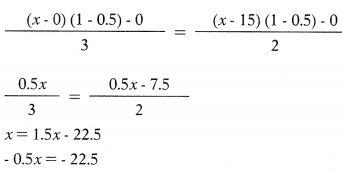x = EBIT=45 Lakhs
At EBIT of ₹ 45 Lakhs, EPS under both option will be same i.e. ₹ 7.5 per share.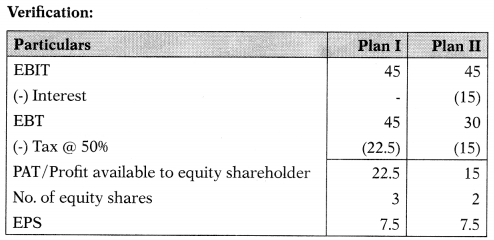Question 91.
Capital structure of A Ltd. is as follows:?Company earns 12% on its employed capital. Tax rate is 35%. It requires a sum of ₹ 25 lakhs to finance its expansion programme for which following plans are available to it:
(i) Issue 20,000 equity shares of ₹ 100 at a premium of ₹ 25 per share or
(ii) Issue 10% preference shares. Calculate indifference point.
(A) EBIT = ₹ 11,21,154
(B) EBIT = ₹ 15,75,154
(C) EBIT = ₹ 16,75,000
(D) EBIT = ₹ 16,00,000
(C) EBIT = ₹ 16,75,000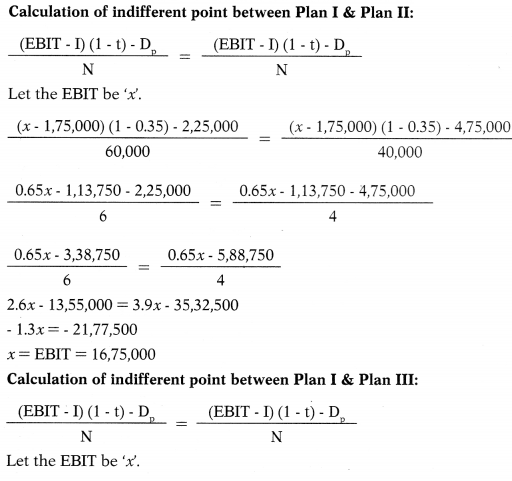Question 92.
EBIT is of NS Ltd. ₹ 4,50,000.
Debt in capital structure = ₹ 7,50,000
Cost of debt (Kd) =11%
Cost of equity (Ke) = 13.5%
Ignore taxation.
Total market value and overall cost of capital of X Ltd. = ?
(A) 34,72,222; 14.96%
(B) 34,27,222;12.96%
(C) 34,72,222; 12.96%
(D) 32,72,222; 14.96%
(C) 34,72,222; 12.96%Analysis: Capital structure having debts of 4,50,000 is recommended as overall cost of capital is minimum.

Question 93.
Capital structure of Z Ltd. is as follows:Company earns 12% onits employed capital. Tax rate is 35%. It requires a sum of ₹ 25 lakhs to finance its expansion programme for which following plans are available to it:
(i) Issue 20,000 equity shares of ₹ 100
at a premium of ₹ 25 per share or
(ii) Issue 8% debentures.
What should be EBIT of the company so that EPS under both plans will be same?
(A) ₹ 11, 12, 154
(B) ₹ 11, 21, 514
(C) ₹ 11, 21, 154
(D) ₹ 11, 21, 4 15
(C) ₹ 11, 21, 154Question 94.
X Ltd. is considering the following two alternative financing plans: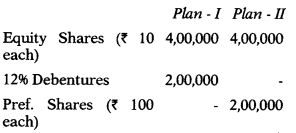The indifference point between the plans is ₹ 2,40,000. Corporate tax rate is 30%. Calculate rate of dividend on preference shares.
(A) 8.00%
(B) 8.04%
(C) 8.40%
(D) 8.80%
(C) 8.40%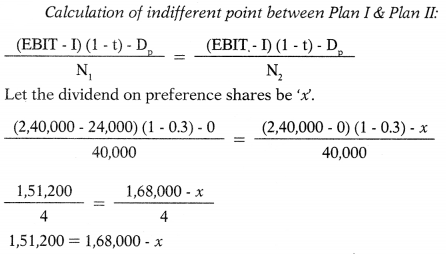Question 95.
EBIT is of NS Ltd. ₹ 4,50,000. Which of the following capital structure will you recommend?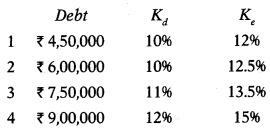Select the correct answer from the options given below:
(A) 1
(B) 2
(C) 3
(D) 4
(A) 1Analysis: Capital structure having debts of 4,50,000 is recommended as overall cost of capital is minimum.

Question 96.
Operating income of A Ltd. is ₹ 5,00,000. The firms cost of debt is 10% and currently firm employs ₹ 15,00,000 of debt. The overall cost of capital of the firm is 15%. You are required to determine ‘total value of the firm’ and ‘market value of equity’ using Net Operating Income Approach (NOI). Ignore taxation.
(A) ₹ 33,33,333; ₹ 15,00,000
(B) ₹ 33,33,333; ₹ 18,33,333
(C) ₹ 18,33,333; ₹ 15,00,000
(D) ₹ 20,22,222; ₹ 18,22,222
(B) ₹ 33,33,333; ₹ 18,33,333

Question 97.
Sun Ltd. has 12% debt of ₹ 30,00,000. It earns 24% before interest and tax on its total assets of ₹ 50,00,000. Tax rate is 40% and capitalization rate is 18%. Calculate the value of the company using Net Income Approach.
(A) ₹ 58,00,000
(B) ₹ 20,00,000
(C) ₹ 55,00,000
(D) ₹ 40,00,000
(B) ₹ 20,00,000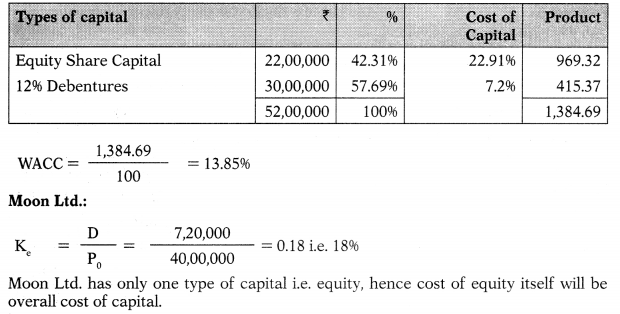Question 98.
Moon Ltd. earns 24% before interest and tax on its total assets of ₹ 50,00,000. It is unlevered company and has no debts in its capital structure. Tax rate is 40% and capitalization rate is 18%. Calculate the value of the company using Net Income Approach.
(A) ₹ 60,00,000
(B) ₹ 40,00,000
(C) ₹ 50,00,000
(D) ₹ 55,00,000
(B) ₹ 40,00,000Question 99.
Sun Ltd. has 12% debt of ₹ 30,00,000. It earns 24% before interest and tax on its total assets of ₹ 50,00,000. Tax rate is 40% and capitalization rate is 18%. Calculate the value of the company using Net Operating Income Approach.
(A) ₹ 58,00,000
(B) ₹ 56,00,000
(C) ₹ 54,00,000
(D) ₹ 52,00,000
(D) ₹ 52,00,000Question 100.
Moon Ltd. earns 24% before interest and tax on its total assets of ₹ 50,00,000. It is unlevered company and has no debts in its capital structure. Tax rate is 40% and capitalization rate is 18%. Calculate the value of the company using Net Operating Income Approach.
(A)  ₹ 20,00,000
(B) ₹ 30,00,000
(C) ₹ 40,00,000
(D) ₹ 50,00,000
(B) ₹ 30,00,000Question 101.
Merry Ltd. has EBIT of ₹ 30,00,000 and 40% tax rate. It required rate of return on equity in the absence of borrowing is 18%. In the absence of personal taxes, what is the ‘total value of the company’ and ‘value of equity’ in an MM world with ₹ 40,00,000 in debt.
(A) ₹ 116 lakh; ₹ 46 lakh
(B) ₹ 116 lakh; ₹ 76 lakh
(C) ₹ 161 lakh; ₹ 67 lakh
(D) ₹ 161 lakh; ₹ 64 lakh
(B) ₹ 116 lakh; ₹ 76 lakh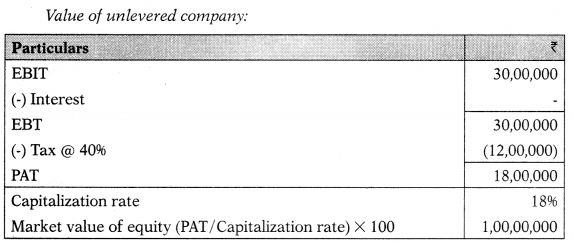According to MM, the value of levered firm would exceed that of the unlevered firm by an amount equal to the levered firms debt multiplied by the tax rate.
Value of unlevered firm + (Value of debt × Tax rate) Total Value of levered firm
Value of the company with 40,00,000 in debt:
1,00,00,000 + (40,00,000 × 40%) = 1,16,00,000
Total value – Value of debt Value of equity
1,16,00,000 – 40,00,000 = 76,00,000
Value of the company with 7000,000 in debt:
1,00,00,000 + (70,00,000 × 40%) = 1,28,00,000
Total value – Value of debt = Value of equity
1,28,00,000 – 70,00,000= 58,00,000

Question 102.
X Ltd. has EBIT of ₹ 30,00,000 and 40% tax rate. It required rate of return on equity in the absence of borrowing is 18%. In the absence of personal taxes, what is the ‘total value of the company’ and ‘value of equity’ in an MM world with ₹ 70,00,000 in debt.
(A) ₹ 128 lakh; ₹ 85 lakh
(B) ₹ 128 lakh; ₹ 58 lakh
(C) ₹ 182 lakh; ₹ 58 lakh
(D) ₹ 182 lakh; ₹ 85 lakh
(B) ₹ 128 lakh; ₹ 58 lakhAccording to MM, the value of levered firm would exceed that of the unlevered firm by an amount equal to the levered firms debt multiplied by the tax rate.
Value of unlevered firm + (Value of debt × Tax rate) Total Value of levered firm
Value of the company with 40,00,000 in debt:
1,00,00,000 + (40,00,000 × 40%) = 1,16,00,000
Total value – Value of debt Value of equity
1,16,00,000 – 40,00,000 = 76,00,000
Value of the company with 7000,000 in debt:
1,00,00,000 + (70,00,000 × 40%) = 1,28,00,000
Total value – Value of debt = Value of equity
1,28,00,000 – 70,00,000= 58,00,000

Question 103.
Following details are presented by Y Ltd.:Company earns a profit of ₹ 3,00,000 per annum after meeting its interest liability of ₹ 1,20,000 on 12% Debentures. Calculate return on capital employed.
(A) 10%
(B) 12%
(C) 14%
(D) 16%
(C) 14%
EBIT = ₹ 3,00,000 + ₹ 1,20,000 = ₹ 4,20,000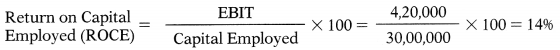Question 104.
Financing alternatives for obtaining the requisite amount of ₹ 20 Crores are under consideration. It was decided to issue equity shares of ₹ 10 par at a premium of 140 each. Share issue expenses as also under pricing of the issue in comparison to ruling market price result in net proceeds of ₹ 40 for every new share issued. How many equity shares are required to be issued for availing finance of ₹ 20 Crore
(A) 0.5 Crore shares
(B) 0.05 Crore shares
(C) 5 Crore shares
(D) 4 Crore shares
(A) 0.5 Crore shares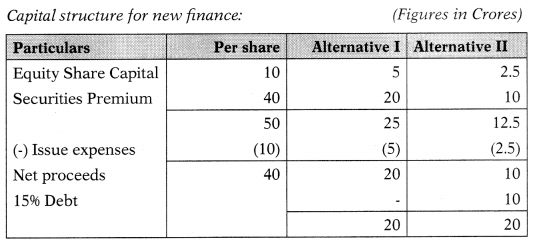Question 105.
Financing alternatives for obtaining the requisite amount of ₹ 20 Crores are under consideration.
Alternative I: Equity shares can be issued at ₹ 10 par with a premium of ₹ 40 each. Share issue expenses as also under pricing of the issue in comparison to ruling market price result in net proceeds of ₹ 40 for every new share issued.
Alternative II: Company can borrow the requisite amount at 15% rate of interest per year.
The company decided to borrow ₹ 10 Crore at 15% rate of interest per year and the balance amount obtained by share issue at par terms indicated in the first alternative.
How many equity shares are required to be issued for availing finance of ₹ 20 Crore?
(A) 0.25 Crore shares
(B) 0.025 Crore shares
(C) 2.5 Crore shares
(D) 25 Crore shares
(A) 0.25 Crore sharesQuestion 106.
A company needs ₹ 31,25,000 for the construction of new plant. The following two plans are feasible:
(i) Company may issue 3,12,500 equity shares at ₹ 10 per share.
(ii) Company may issue 1,56,250ordinary equity shares at ₹ 10 per share and 15,625 debentures of ₹ 100 denomination bearing an 8% rate of interest.
Corporate income-tax rate is 40%. Calculate indifference point.
(A) ₹ 2,50,000
(B) ₹ 2,75,000
(C) ₹ 2,25,000
(D) ₹ 3,00,000
(A) ₹ 2,50,000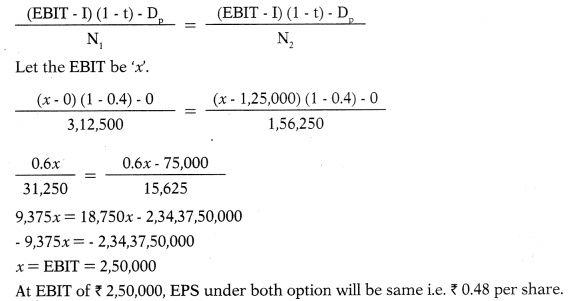Question 107.
A company needs ₹ 31,25,000 for the construction of new plant. The following two plans are feasible:
(i) Company may issue 3,12,500 equity shares at ₹ 10 per share.
(ii) Company may issue 1,56,250 equity shares at ₹ 10 per share and 15,625 preference shares at ₹ 100 per share bearing an 8% rate of dividend.
Corporate income-tax rate is 40%.
At indifferent point EPS under both plans will be -…….
(A) ₹ 0.75 per share
(B) ₹ 0.95 per share
(C) ₹ 0.60 per share
(D) ₹ 0.80 per share
(D) ₹ 0.80 per share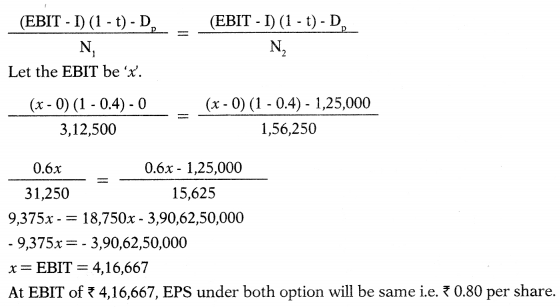Question 108.
M Ltd. requires ₹ 25,00,000 for a new plant. This plant is expected to yield EBIT of ₹ 5,00,000. The company considers the objectives of maximizing EPS. It has 3 options to finance the project – by raising debt of ₹ 2,50,000 or ₹ 10,00,000 or ₹ 15,00,000 and the balance, in each case, by issuing equity shares. Company’s shares is currently selling at ₹ 150, but it is expected to decline to ₹ 125 in case the funds are borrowed in excess of ₹ 10,00,000. The funds can be borrowed at the rate of 10% up to ₹ 2,50,000 and 15% up to ₹ 10,00,000 and at 20% over ₹ 10,00,000. The tax rate is 50%. Which form of financing should company choose?
(A) Option III containing debt issue of ₹ 15,00,000
(B) Option II containing debt issue of ₹ 10,00,000
(C) Option II containing debt issue of ₹ 2,50,000
(D) None of the above
(B) Option II containing debt issue of ₹ 10,00,000

Question 109.
Domino is an all equity firm with a current cost of equity of 18%. The EBIT of the firm is ₹ 2,04,000 annually forever. Currently, the firm has no debt but is in the process of borrowing ₹ 5,00,000 at 9% interest. The tax rate is 34%. What is the value of the unlevered firm?
(A) ₹ 7,23,150
(B) ₹ 6,54,900
(C) ₹ 7,48,000
(D) ₹ 6,09,900
(C) ₹ 7,48,000Question 110.
There are two firms P and Q which are identical except P does not use any debt in its capital structure while Q has ₹ 8,00,000, 9% debentures in its capital structure. Both the firms have EBIT of ₹ 2,60,000 p.a. and the capitalization rate is 10%. Corporate tax is 30%. Calculate the total market value of these firms according to MM Hypothesis.
(A) ₹ 20,60,000; ₹ 18,20,000
(B) ₹ 18,20,000; ₹ 20,60,000
(C) ₹ 18,20,000; ₹ 12,60,000
(D) ₹ 12,60,000; ₹ 20,60,000
(B) ₹ 18,20,000; ₹ 20,60,000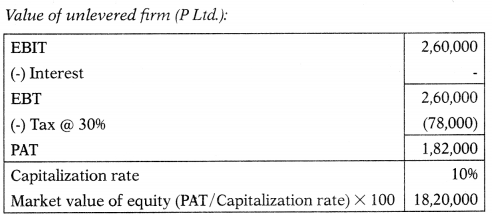According to MM, the value of levered firm would exceed that of the unlevered firm by an amount equal to the levered firms debt multiplied by the tax rate.
Value of unlevered firm + (Value of debt × Tax rate) = Total Value
Value of the company with 8,00,000 In debt (Q Ltd.):
18,20,000 + (8,00,000 × 30%) 20,60,000
Total value – Value of debt Value of equity
20,60,000 – 8,00,000 = 12,60,000

Question 111.
B Ltd. requires ₹ 12 lakh to finance its activities. Its earnings before interest and tax amount to ₹ 2 lakh. The Finance Manager has forwarded three proposals:The market price of a share of the company is 40 which is expected to come down to 25 a share, if the market borrowings exceeds 7,50,000. Which proposal is most profitable proposal from shareholders point view?
(A) Proposal I
(B) Proposal II
(C) Proposal Ill
(D) None of the above
(B) Proposal IIInterest expenses for various proposals:
Proposal-I 2,00,000 × 10% = 20,000
Proposal-II = (2,50,000 × 10%) + (3,50,000 × 14%) = 74,000
Proposal-III = (2,50,000 × 10%) + (3,75,000 × 14%) + (3,75,000 × 16%) = 1,37,500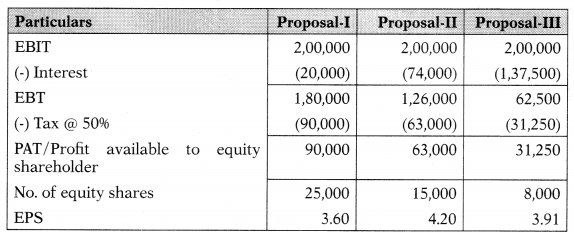Analysis: Since, EPS is highest under Proposal-il, the company is advised to go with this proposal.

Question 112.
Skyline Software Ltd. wants to implement a project for which 30 lakhs is required. Following financing options are at hand:
Option 1:
Equity Shares — 30,000
Option 2
Equity Shares — 10,000
12% Preference Shares — 10,000
10% Debentures — 10,000
Calculate the indifference point & EPS at that level of EBIT assuming corporate tax to be 35%.
(A) ₹ 4,26,923; ₹ 12.00
(B) ₹ 4,26,923; ₹ 9.25
(C) ₹ 5,53,846; ₹ 9.25
(D) ₹ 3,00,000; ₹ 6.25
(B) ₹ 4,26,923; ₹ 9.25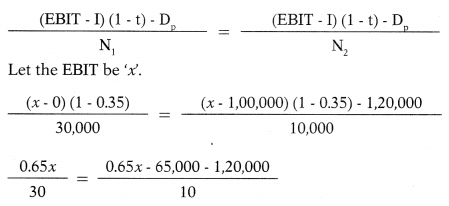6.5x = 19.5x – 55,50ß00
– 13x = – 5550,000
x = EBIT = 4,26,923
At EBIT of ₹ 4,26,923, EPS under both option will be same i.e ₹ 9.25 per share.

Question 113.
Alpha Ltd. is contemplating conversion of 500 14% convertible bonds of ₹ 1,000 each. Market price of the bond is ₹ 1,080. Bond indenture provided that 1 bond will be exchanged for 10 shares. P/E ratio before redemption is 20:1 and anticipating price earnings ratio after redemption is 25:1. Number of shares outstanding prior to redemption are 10,000. EBIT amounts to ₹ 2,00,000. The company is in the 35% tax bracket.
Calculate market price –
(i) Pre-redemption and
(ii) Post redemption.
(A) ₹ 169 & ₹ 216.67 per share
(B) ₹ 159 & ₹ 206.67 per share
(C) ₹ 179 & ₹ 226.67 per share
(D) ₹ 170 & ₹ 220 per share
(A) ₹ 169 & ₹ 216.67 per share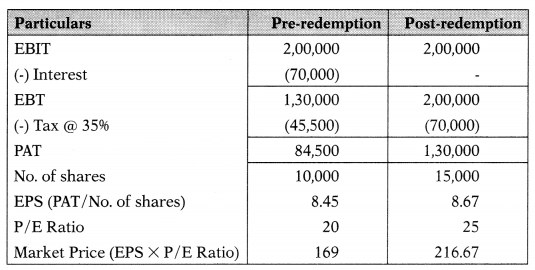Question 114.
Following data is available for Company X:
Total Assets : ₹ 15,00,000
1096 Debt : ₹ 9,00,000
EBIT : 2096 of total assets
Tax rate : 50%
Capitalization rate =15%
Calculate market value of equity using Net Income (NT) approach.
(A) ₹ 16,00,000
(B) ₹ 7,00,000
(C) ₹ 10,00,000
(D) ₹ 5,00,000
(B) ₹ 7,00,000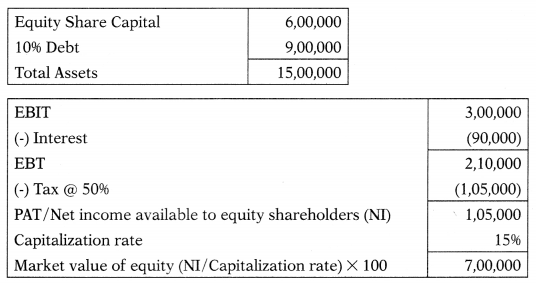Question 115.
Following data is available for Company Y:
Total Assets : ₹ 15,00,000
Debt : Nil
EBIT : 20% of total assets
Tax rate : 50%
Capitalization rate =15%
Calculate market value of equity using Net Income (NI) approach.
(A) ₹ 10,00,000
(B) ₹ 16,00,000
(C) ₹ 12,00,000
(D) ₹ 15,00,000
(A) ₹ 10,00,000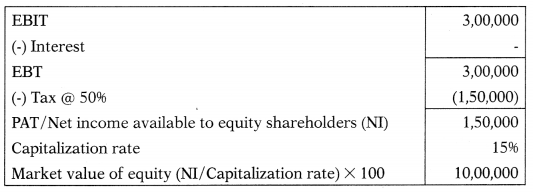Question 116.
Following data is available for P Ltd.:
Total Assets : ₹ 15,00,000
1096 Debt : ₹ 9,00,000
EBIT : 20% of total assets
Tax rate : 50%
Capitalization rate =15%
Calculate total value of the firm using Net Operating Income (NOI) approach.
(A) ₹ 5,50,000
(B) ₹ 14,50,000
(C) ₹ 10,50,000
(D) ₹ 12,50,000
(B) ₹ 14,50,000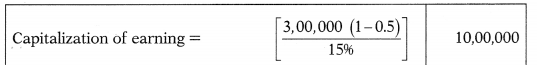Question 117.
Following data is available for Q Ltd.:
Total Assets : ₹ 15,00,000
Debt : Nil
EBIT : 20% of total assets
Tax rate : 50%
Capitalization rate =15%
Calculate total value of the firm using Net Operating Income (NOI) approach.
(A) ₹ 8,00,000
(B) ₹ 9,00,000
(C) ₹ 12,00,000
(D) ₹ 10,00,000
(D) ₹ 10,00,000

Question 118.
A student studying Financial Management subject is not able to understand when total market value will be the same for Company X and Company Y if both companies have same total assets.
Company X calculates total value under Net Income (NI) approach and Company Y calculates total value under Net Operating
Income (NOI) approach. Help him by selecting correct option.
(A) When tax rate is same for both the companies.
(B) When one company is totally financed by debt and other is totally finance by equity.
(C) When both companies are financed by equity only.
(D) When both companies adopt same selling price.
(C) When both companies are financed by equity only.

Question 119.
Trade International is an all-equity firm that has projected earnings before interest and taxes of ₹ 4,97,000 forever. The current cost of equity is 16% and the tax rate is 34%. The company is in the process of issuing ₹ 1.5 million of bonds at par that carry a 6% annual coupon. What is the levered value of the firm?
(A) ₹ 20, 50, 125
(B) ₹ 24, 48, 009
(C) ₹ 21, 13, 609
(D) ₹ 25, 60, 125
(D) ₹ 25, 60, 125According to MM, the value of levered firm would exceed that of the unlevered firm by an amount equal to the levered firms debt multiplied by the tax rate.
Value of unlevered firm + (Value of debt × Tax rate) = Total Value Value of the company with 15,00,000 In debt:
20,50,125 + (15,00,000 × 34%) = 25,60,125

Question 120.
Bharat Cylinders has 15,000 shares of stock outstanding and no debt, as the original founder of the firm did not approve of debt financing. The new CEO is considering issuing ₹ 2,50,000 of debt and using the proceeds to retire 5,000 shares of stock. The interest rate on debt is 7.5%. What is the break-even level of EBIT between these two capital structure options?
(A) ₹ 59,250
(B) ₹ 38,500
(C) ₹ 56,250
(D) ₹ 47,750
(C) ₹ 56,250Question 121.
What is the market value of common equity under the NOI approach? The firm has an expected net operating income of ₹ 5,000 with ₹ 4,000 of debt (market value). Assume that the overall capitalization rate is 20%.
(A) ₹ 5,000
(B) ₹ 20,000
(C) ₹ 21,000
(D) ₹ 24,000
(C) ₹ 21,000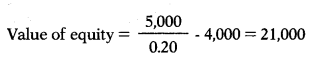Question 122.
Ganesha Ltd. is setting up a project with a capital outlay of ₹ 60,00,000. It has two alternatives in financing the project cost.
Alternative (a): 100% equity finance
Alternative (b): Debt-equity ratio 2:1
The rate of interest payable on the debts is 18% p.a. Corporate tax rate is 40%. Calculate the indifference point between the two alternative methods of financing.
(A) ₹ 10,80,000
(B) ₹ 18,80,000
(C) ₹ 18,00,000
(D) ₹ 10,08,000
(A) ₹ 10,80,000

Question 123.
One-third of the total market value of X Ltd. consists of loan stock, which has a cost of 10%. Another company, Y Ltd. is identical in every respect to X Ltd., except that its capital structure is all-equity, and its cost of equity is 16%. According to Modigliani and Miller, if we ignored taxation and tax relief on debt capital, what would be the cost of equity of X Ltd.?
(A) 14%
(B) 17%
(C) 19%
(D) 13%
(C) 19%
Miller and Modigliani’s first model argues that no optimal capital structure exists and supports this proposition with arbitrage theory. Therefore, the two companies should have similar WACCs. Because Y Ltd. is all-equity financed, its WACC is the same as its cost of equity finance, i.e. 16%. It follows that X Ltd. should have
WACC equal to 16% also.
Therefore, (1/3 × 10%) + (2/3 × Ke) 16%
3.3333 + 0.6667x =16
06667x = 12.6667
Hence, x = Ke = 19%.

Question 124.
IPL Ltd. has EBIT of ₹ 1,00,000. The company makes use of debt and equity capital. The firm has 10% debentures of ₹ 5,00,000 and the firm’s equity capitalization rate is 15%. You are required to compute: (i) Current value of the firm; (ii) Overall cost of capital.
(A) ₹ 3,33,333; 15%
(B) ₹ 8,33,333; 12%
(C) ₹ 5,33,333; 14%
(D) ₹ 6,33,333; 18%
From the following information answer next 4 questions.
Alpha Ltd. & Beta Ltd. are identical except for capital structures. Alpha has 50% debt and 50% equity, whereas Beta has 20% debt and 80% equity. All percentages are in market-value terms. The borrowing rate for both companies is 8% in a no-tax world, and capital markets Eire assumed to be perfect. Both companies have net operating income of ₹ 3,60,000 and the overall capitalization rate of the company, Ke is 18%.
(B) ₹ 8,33,333; 12%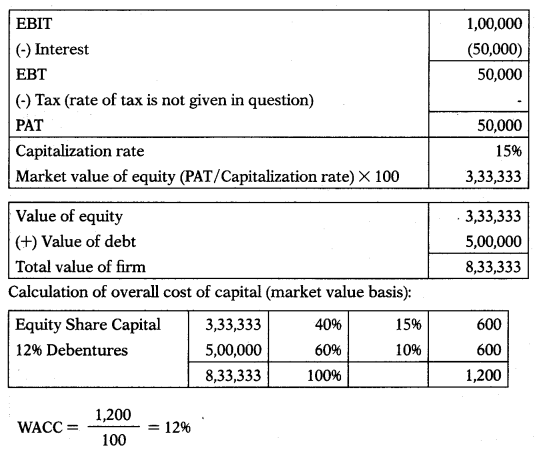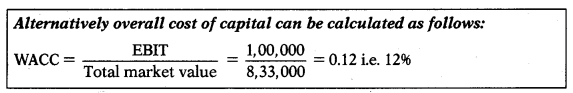This method to calculate overall cost of capital works i/there is no tax. If tax rate is given in question then overall cost of capital Le. WACC should be calculated as per method shown above.

Question 125.
If you own 2% of the stock of Alpha, what is your return?
(A) ₹ 5,000
(B) ₹ 4,600
(C) ₹ 3,500
(D) ₹ 5,600
(D) ₹ 5,600Implied required equity return (Beta Ltd.) =$$\frac{3,28,000}{10,00,000}=20.5 \%$$

Question 126.
What is the implied required rate of return on equity of Alpha Ltd.?
(A) 22%
(B) 26%
(C) 20%
(D) 28%
(D) 28%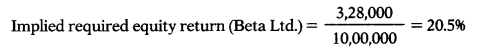Question 127.
What is the market value of equity of Beta Ltd.?
(A) ₹ 16,00,000
(B) ₹ 14,00,000
(C) ₹ 12,00,000
(D) ₹ 10,00,000
(A) ₹ 16,00,000Question 128.
What is the implied required rate of return on equity of Beta Ltd.?
(A) 10.5%
(B) 18,5%
(C) 15.5%
(D) 20.5%
From the following information answer next 4 questions.
RES Ltd. is an all equity financed company with a market value of ₹ 25,00,000 and cost of equity, Ke = 21%. The company wants to buy back equity shares worth ₹ 5,00,000 by issuing and raising 15% perpetual debt of the same amount. Rate of tax may be taken as 30%. After the capital restructuring and applying MM Model (with taxes), you are required to answer next 2 questions:
(D) 20.5%Question 129.
What is the EBIT of the unlevered firm?
(A) ₹ 5,25,000
(B) ₹ 6,70,000
(C) ₹ 7,50,000
(D) ₹ 6,50,000
(C) ₹ 7,50,000Question 130.
What is the PAT of levered firm?
(A) ₹ 5,25,000
(B) ₹ 4,52,700
(C) ₹ 4,72,500
(D) ₹ 4,25,700
(C) ₹ 4,72,500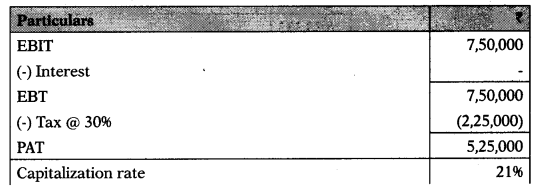According to MM, the value of levered firm would exceed that of the unlevered firm by an amount equal to the levered firms debt multiplied b’ the tax rate.
Value of unlevered firm + (Value of debt × Tax rate) = Total Value
Value of the company with 5,00,000 In debt:
25,00,000 + (5,00,000 × 30%) 26,50,000
Total value – Value of debt = Value of equity
26,50,000 – 5,00,000 = 21,50,000
$$\mathrm{K}_{e}=\frac{D}{P_{0}}=\frac{4,72,500}{21,50,000}=0.2198 \text { i.e. } 21.98 \%$$
Cost of debt:
Kd =I(1 -t)
Ki = 15(1 -0.30)
Ki = 10.5%
Calculation of overall cost of capital (market value basis):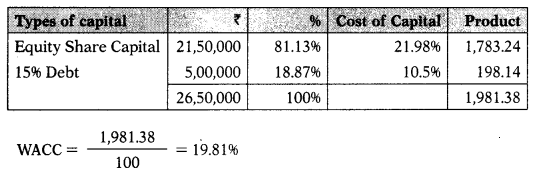Question 131.
What is the value of equity of levered firm?
(A) ₹ 26,50,000
(B) ₹ 22,50,000
(C) ₹ 21,50,000
(D) ₹ 24,50,000